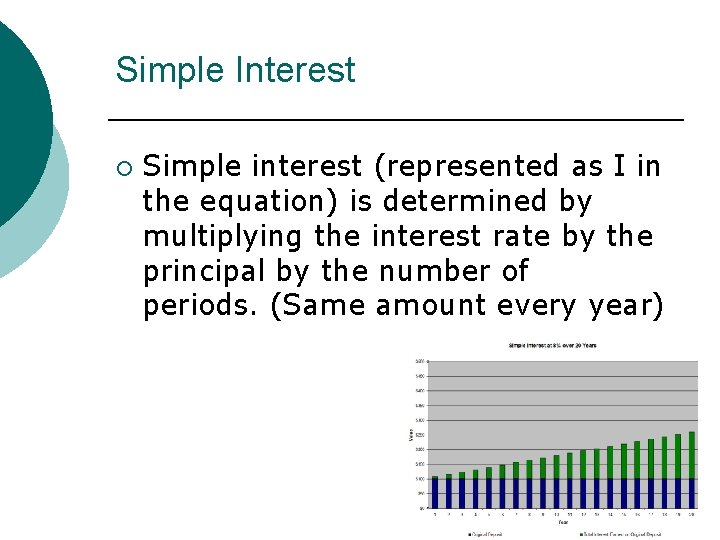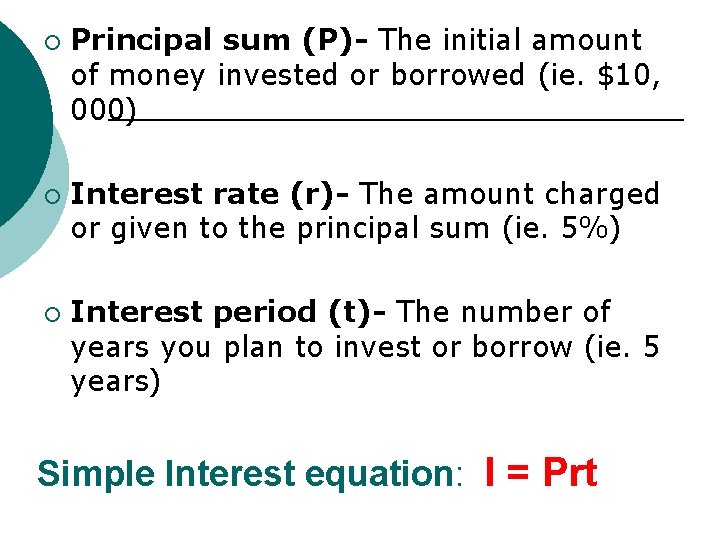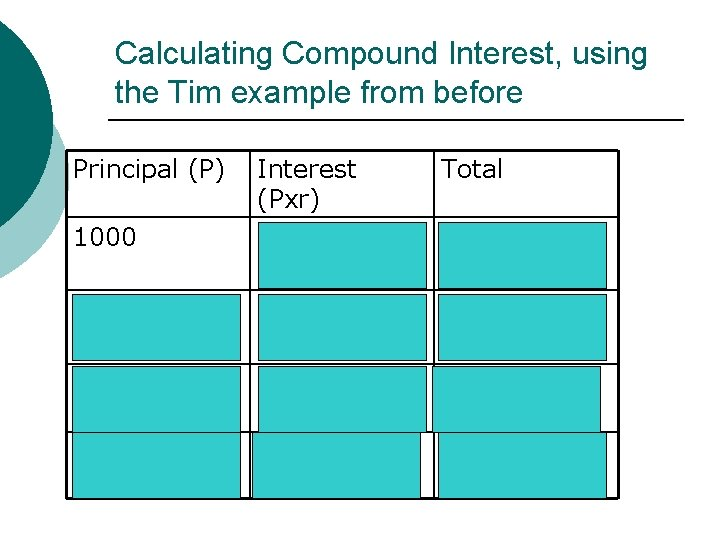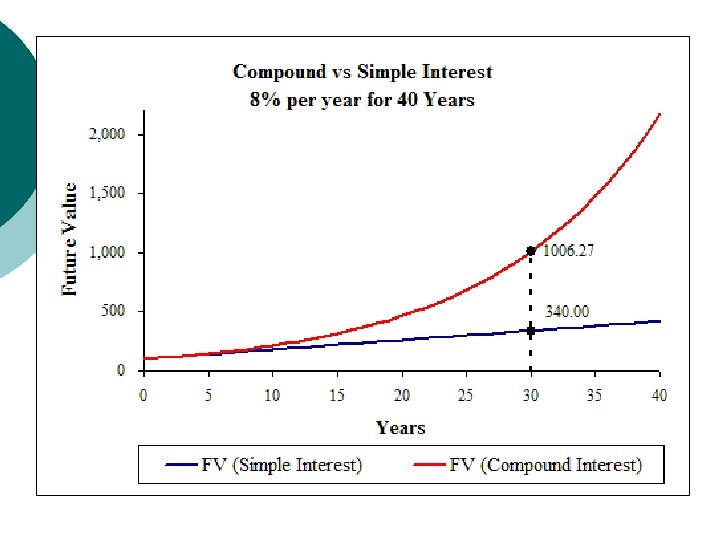# Calculating Simple Compound Interest Simple Interest Simple interest

• Slides: 8Calculating Simple & Compound InterestSimple Interest ¡ Simple interest (represented as I in the equation) is determined by multiplying the interest rate by the principal by the number of periods. (Same amount every year)¡ ¡ ¡ Principal sum (P)- The initial amount of money invested or borrowed (ie. \$10, 000) Interest rate (r)- The amount charged or given to the principal sum (ie. 5%) Interest period (t)- The number of years you plan to invest or borrow (ie. 5 years) Simple Interest equation: I = PrtLet’s try it Tim, a grade 9 student at Preston High school received \$1000 from his grandma for his birthday. After learning about savings in his BBI class, he decides to go to his bank and put the money into a savings account at an interest rate of 3% annually until he goes to university in 4 years. Calculate the simple interesting using the simple interest equation: I=PrtI = Prt P = \$1000 ¡ r= 3% annually ¡ t= 4 years ¡ I = 1000 x 0. 03 x 4 = 120 Therefore Tim will have made \$120 in interest over the 4 years resulting in a total of \$1120.Compound Interest ¡ Interest calculated on the amount saved or borrowed plus any interest already accumulatedCalculating Compound Interest, using the Tim example from before Principal (P) 1000 1030 1060. 90 Interest (Pxr) = 1000 x. 03 = 30 = 1030 x. 03 = 30. 90 =1060. 90 x. 03 Total = 1030 = 1060. 90 = 1092. 73 = 31. 83 1092. 73 = 1092. 73 x. 03 = 1125. 51 = 32. 78